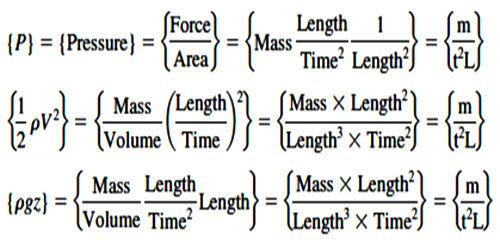# Principle of Homogeneity of Dimensions

An equation is dimensionally correct if the dimensions of the various terms on either side of the equation are the same. This is called the principle of homogeneity of dimensions. This principle is based on the fact that two quantities of the same dimension only can be added up, the resulting quantity also possessing the same dimension.

The equation A + B = C is valid only if the dimensions of A. B and C are the same.

Dimensional analysis is a very helpful instrument in Physics for finding out and verifying the relationship between diverse quantities that go into making a phenomenon. Any equation or formula involving dimensions, like mass, length, time, and temperature electricity, etc. have the terms with similar dimensions. This helps us, therefore, to convert the units in one system to another system and also helps us to check a formula or the involvement of the dimensions in a formula.The principle of homogeneity of dimensions states that “For an equation to be dimensionally correct, the dimensions of each term on “Left Hand Side” must be equivalent to the dimensions of each term on “Right Hand Side.” So, it states that if the dimensions of each term on both the sides of the equation are similar, then the physical measurement will be accurate.

Examples, to check the correctness of v = u + at, using dimensions

• Dimensional formula of final velocity, v = [LT-1]
• Dimensional formula of final velocity, u = [LT-1]
• Dimensional formula of acceleration x time, at = [LT-2 × T] = [LT-1]

When a physical equation consists of a number of terms, each of these terms must be of similar dimensions in each of the primary units. Here, Dimensions on both sides of each term are the same. Hence, the equation is dimensionally correct.

Dimensional Analysis Applications –

1. To determine the dimensions of an unknown quantity in an equation
2. To check the accuracy of an equation, sometimes we might be required either to derive or to check a specific relation.
3. To derive a formula connecting the given physical quantities and convert a physical quantity from one system of a unit to other

Thus, dimensional analysis is the process of analyzing a physical problem with the help of dimensional equations.

Following are the main uses of dimensional analysis –

The method of dimensional analysis is used to:

(a) Checking the dimensional correctness of a physical equation. If the dimensions follow the principle of homogeneity, the equation is dimensionally approved, otherwise not.

(b) Deriving the relationship between different physical quantities; in many cases, we can find the expression for a physical quantity, if we know the factors on which it depends.

(c) Conversion of one system of units into another. This fact helps us to change one system of units into another.

(d) Convert a physical quantity from one system of units to another.

(e) Check the dimensional correctness of a given equation.

(f) Establish a relationship between different physical quantities in an equation.